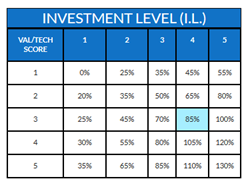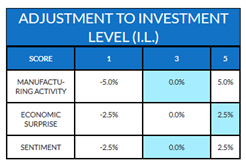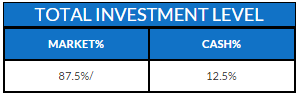# Strategy – Investment Level – ENThis module is the reconciliation of all the analysis computed in the "strategy" part: based on the different analysis (technical analysis, sentiment, macro, valuation, ...), we aggregate the different results and determine an optimal investment level (allocation equities vs cash).

Sigma-Analytics doesn’t consider it is appropriate to be always fully invested. Indeed, equity markets are driven by their valuation level, their momentum, the monetary policy, ….
So, we built our own investment matrix based on 2 main factors: the valuation level (as analysed in Strategy -> Valuation) and the technical level (as analysed in Strategy -> Technical Analysis). Based on this matrix, we compute the core investment level. When equity market is evolving in a moderate uptrend (technical score = 4) with neutral valuation (this means neither cheap nor expensive-> valuation score = 3), our core investment level is 85% invested in equities and 15% in cash.Then, based on manufacturing activity, investors’ sentiment and economic surprise, we apply some deviations to this core investment level in order to compute our final investment level.
For example, if manufacturing activity is neutral (score = 3), economic surprises were highly negative and give some signs of normalization (score = 5) and investors’ sentiment is neutral (which means that bulls/bears spread is normal -> score = 3), then we will apply following deviations:And our total investment level increases from 85% to 87.5%: